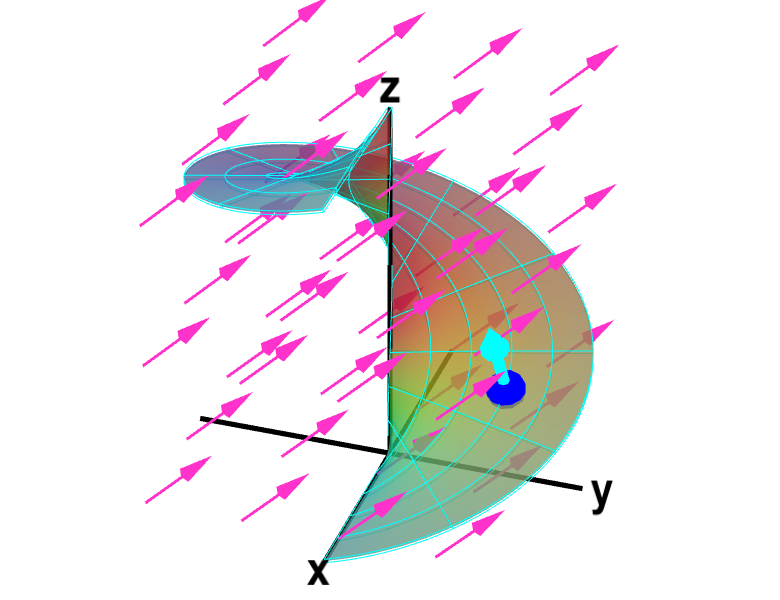# Math Insight

### Applet: Fluid flow through oriented helicoidThe function $\dlsp(\spfv,\spsv) = (\spfv\cos \spsv, \spfv\sin \spsv, \spsv)$ parametrizes a helicoid when $(\spfv,\spsv) \in \dlr$, where $\dlr$ is the rectangle $[0,1] \times [0, 2\pi]$ shown in the first panel. The cyan vector at the blue point $\dlsp(\spfv,\spsv)$ is the upward pointing unit normal vector at that point. The magenta vector field represents fluid flow that passes through the surface. In this example, the vector field is the constant $\dlvf=(0,1,1)$. You can drag the blue point in $\dlr$ or on the helicoid to specify both $\spfv$ and $\spsv$.